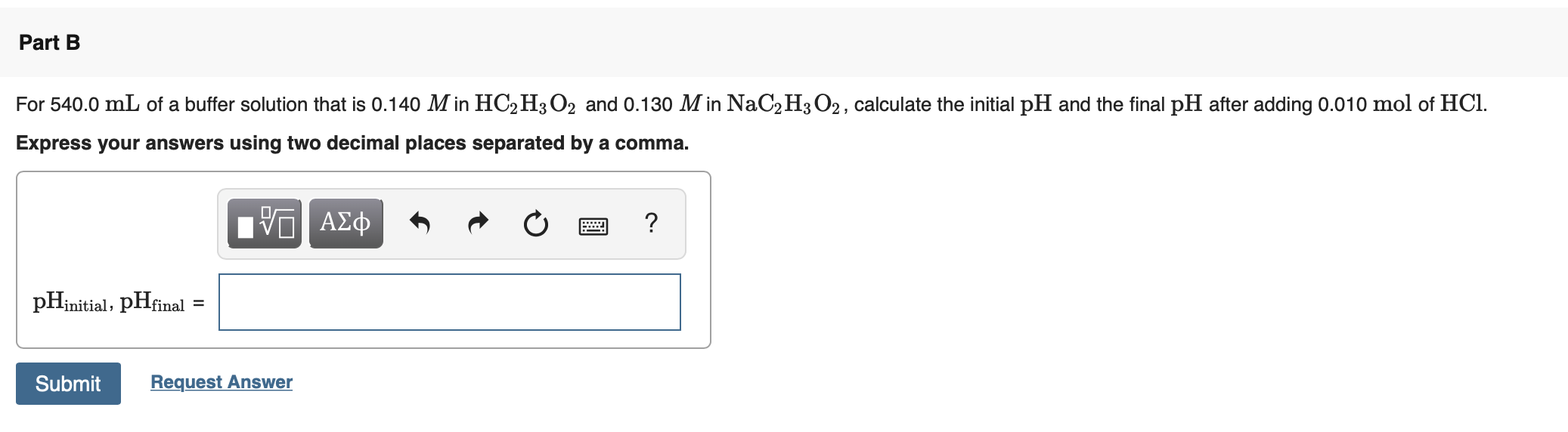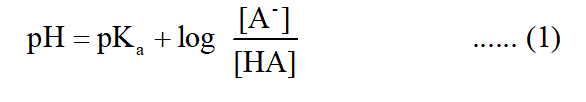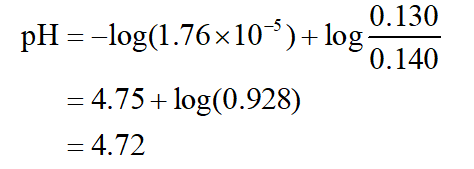# Part BFor 540.0 mL of a buffer solution that is 0.140 M in HC2 H3O2 and 0.130 M in NaC2H3 O2, calculate the initial pH and the final pH after adding 0.010 mol of HCl.Express your answers using two decimal places separated by a comma.μνα ΑΣφpHinitial, pHfinal =SubmitRequest Answer

Question
1 views

Can someone show me how to answer this problem and the chemical equation of the buffer solution?help_outlineImage TranscriptionclosePart B For 540.0 mL of a buffer solution that is 0.140 M in HC2 H3O2 and 0.130 M in NaC2H3 O2, calculate the initial pH and the final pH after adding 0.010 mol of HCl. Express your answers using two decimal places separated by a comma. μνα ΑΣφ pHinitial, pHfinal = Submit Request Answer fullscreen
check_circle

Step 1

pH of a buffer solution is given by:Ka = 1.76×10-5

Substituting the values in equation (1):Initial pH of the solution = 4.72

...

### Want to see the full answer?

See Solution

#### Want to see this answer and more?

Solutions are written by subject experts who are available 24/7. Questions are typically answered within 1 hour.*

See Solution
*Response times may vary by subject and question.
Tagged in